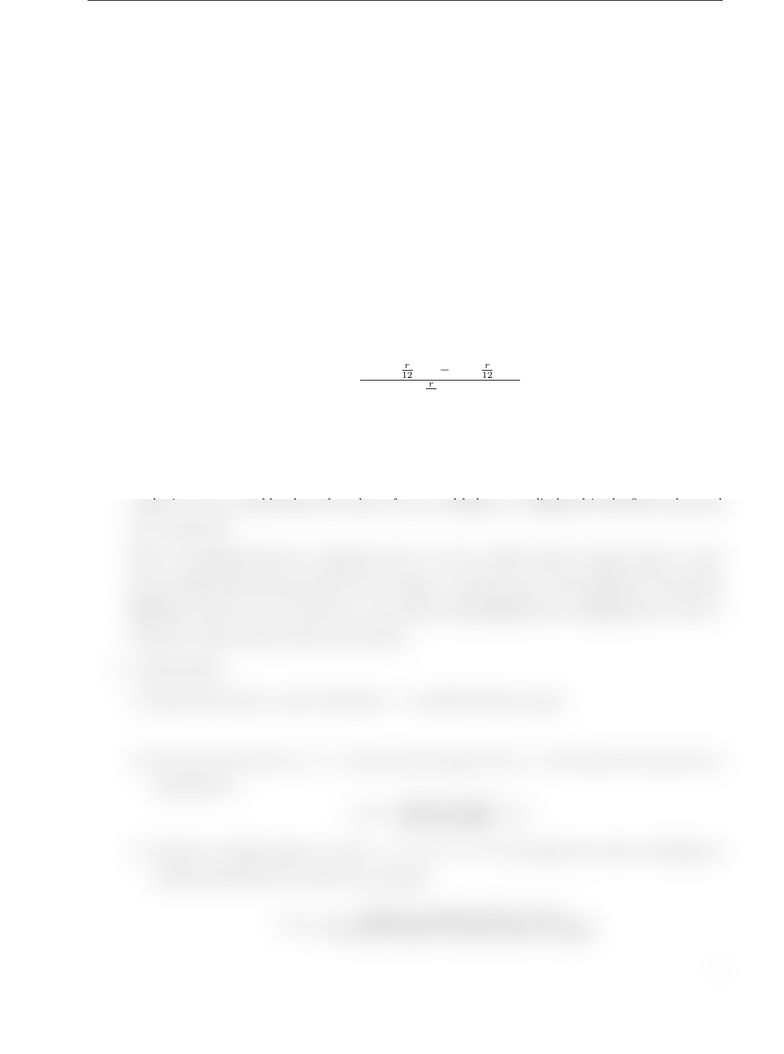Study Guides (400,000)
CA (150,000)
York (10,000)
ITEC (100)
Quiz

# ITEC 2600 Study Guide - Quiz Guide: EllipseExam

Department
Information Technology
Course Code
ITEC 2600
Professor
Xiaofeng Zhou
Study Guide
Quiz

This preview shows half of the first page. to view the full 2 pages of the document.ITEC2600 Winter2018 X.Zhou York University
ITEC 2600
Introduction to Analytical Programming
Lab Exercise 8
1. Redo all examples from the lecture.
2. Write a function that will receive a variable number of input arguments: the semi-major axe(a) and semi-
minor axe (b) of a ellipse, and possibly also the height(h) of elliptic cylinder that has this ellipse as its
base. The function should return the ellipse area if just the length and width are passed(area =π×a×b),
or also the volume(v=π×a×b×h) if the height is also passed.
3. Probelm 7 from Textbook: An Introduction with Application, page 87.
The remaining loan balance, B, of a ﬁxed payment nyears mortgage after xyears is given by:
B=L[(1 + r
12 )12n(1 + r
12 )12x]
(1 + r
12 )12n1
where Lis the loan amount, and ris the annual interest rate. Calculate the balance of a 30-year,
100,000 mortgage, with annual interest rate of 6% (use 0.06 in the equation) after 0, 5, 10, 15, 20, 25,
and 30 years. Create a seven-element vector for xand use element-by-element operations. Display the
results in a two-row table where the values of years and balance are displayed in the rst and second
rows, respectively.
Write a user-dened function to implement above. Use the variable number of input/output to make
this user-dened function more general. For example, a customer want to know dierence of borrowing
200,000 at interest rate 5% and %6, or can customer aﬀord 200,000 loan or 250,000 loan? Write a
script le to help customer make smart decision.
4. πapproximation
(a) Figure below shows a circle with radius r= 1 inscribed within a square.
(b) The area of the circle is πr2=π, the area of the square is (2r) = 4. The ratio of two areas can be
represented as
ratio =Area of circle
Area of square =π
4
(c) Generate a random point(x, y), where 1<=x;y <= 1, we can estimate the ratio by checking the
random points falls in the which area as follows:
ratio =Number of points fall in circle
T he total number of pints f alls in square
1